Skip to content →

# Category: games

In ONAG, John Conway proves that the symmetric version of his recursive definition of addition and multiplcation on the surreal numbers make the class On of all Cantor’s ordinal numbers into an algebraically closed Field of characteristic two : On2 (pronounced ‘Onto’), and, in particular, he identifies a subfield
with the algebraic closure of the field of two elements. What makes all of this somewhat confusing is that Cantor had already defined a (badly behaving) addition, multiplication and exponentiation on ordinal numbers.

Over the last week I’ve been playing a bit with sage to prove a few exotic identities involving ordinal numbers. Here’s one of them ($\omega$ is the first infinite ordinal number, that is, $\omega={ 0,1,2,\ldots }$),

$~(\omega^{\omega^{13}})^{47} = \omega^{\omega^7} + 1$

answering a question in Hendrik Lenstra’s paper Nim multiplication.

However, it will take us a couple of posts before we get there. Let’s begin by trying to explain what brought this on. On september 24th 2008 there was a meeting, intended for a general public, called a la rencontre des dechiffeurs, celebrating the 50th birthday of the IHES.

One of the speakers was Alain Connes and the official title of his talk was “L’ange de la géométrie, le diable de l’algèbre et le corps à un élément” (the angel of geometry, the devil of algebra and the field with one element). Instead, he talked about a seemingly trivial problem : what is the algebraic closure of $\mathbb{F}_2$, the field with two elements? My only information about the actual content of the talk comes from the following YouTube-blurb

Alain argues that we do not have a satisfactory description of $\overline{\mathbb{F}}_2$, the algebraic closure of $\mathbb{F}_2$. Naturally, it is the union (or rather, limit) of all finite fields $\mathbb{F}_{2^n}$, but, there are too many non-canonical choices to make here.

Recall that $\mathbb{F}_{2^k}$ is a subfield of $\mathbb{F}_{2^l}$ if and only if $k$ is a divisor of $l$ and so we would have to take the direct limit over the integers with respect to the divisibility relation… Of course, we can replace this by an increasing sequence of a selection of cofinal fields such as

$\mathbb{F}_{2^{1!}} \subset \mathbb{F}_{2^{2!}} \subset \mathbb{F}_{2^{3!}} \subset \ldots$

But then, there are several such suitable sequences! Another ambiguity comes from the description of $\mathbb{F}_{2^n}$. Clearly it is of the form $\mathbb{F}_2[x]/(f(x))$ where $f(x)$ is a monic irreducible polynomial of degree $n$, but again, there are several such polynomials. An attempt to make a canonical choice of polynomial is to take the ‘first’ suitable one with respect to some natural ordering on the polynomials. This leads to the so called Conway polynomials.

Conway polynomials for the prime $2$ have only been determined up to degree 400-something, so in the increasing sequence above we would already be stuck at the sixth term $\mathbb{F}_{2^{6!}}$…

So, what Alain Connes sets as a problem is to find another, more canonical, description of $\overline{\mathbb{F}}_2$. The problem is not without real-life interest as most finite fields appearing in cryptography or coding theory are subfields of $\overline{\mathbb{F}}_2$.

(My guess is that Alain originally wanted to talk about the action of the Galois group on the roots of unity, which would be the corresponding problem over the field with one element and would explain the title of the talk, but decided against it. If anyone knows what ‘coupling-problem’ he is referring to, please drop a comment.)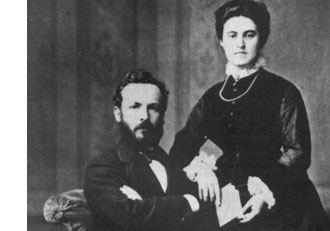Surely, Connes is aware of the fact that there exists a nice canonical recursive construction of $\overline{\mathbb{F}}_2$ due to John Conway, using Georg Cantor’s ordinal numbers.

In fact, in chapter 6 of his book On Numbers And Games, John Conway proves that the symmetric version of his recursive definition of addition and multiplcation on the surreal numbers make the class $\mathbf{On}$ of all Cantor’s ordinal numbers into an algebraically closed Field of characteristic two : $\mathbf{On}_2$ (pronounced ‘Onto’), and, in particular, he identifies a subfield

$\overline{\mathbb{F}}_2 \simeq [ \omega^{\omega^{\omega}} ]$

with the algebraic closure of $\mathbb{F}_2$. What makes all of this somewhat confusing is that Cantor had already defined a (badly behaving) addition, multiplication and exponentiation on ordinal numbers. To distinguish between the Cantor/Conway arithmetics, Conway (and later Lenstra) adopt the convention that any expression between square brackets refers to Cantor-arithmetic and un-squared ones to Conway’s. So, in the description of the algebraic closure just given $[ \omega^{\omega^{\omega}} ]$ is the ordinal defined by Cantor-exponentiation, whereas the exotic identity we started out with refers to Conway’s arithmetic on ordinal numbers.

Let’s recall briefly Cantor’s ordinal arithmetic. An ordinal number $\alpha$ is the order-type of a totally ordered set, that is, if there is an order preserving bijection between two totally ordered sets then they have the same ordinal number (or you might view $\alpha$ itself as a totally ordered set, namely the set of all strictly smaller ordinal numbers, so e.g. $0= \emptyset,1= { 0 },2={ 0,1 },\ldots$).

For two ordinals $\alpha$ and $\beta$, the addition $[\alpha + \beta ]$ is the order-type of the totally ordered set $\alpha \sqcup \beta$ (the disjoint union) ordered compatible with the total orders in $\alpha$ and $\beta$ and such that every element of $\beta$ is strictly greater than any element from $\alpha$. Observe that this definition depends on the order of the two factors. For example,$[1 + \omega] = \omega$ as there is an order preserving bijection ${ \tilde{0},0,1,2,\ldots } \rightarrow { 0,1,2,3,\ldots }$ by $\tilde{0} \mapsto 0,n \mapsto n+1$. However, $\omega \not= [\omega + 1]$ as there can be no order preserving bijection ${ 0,1,2,\ldots } \rightarrow { 0,1,2,\ldots,0_{max} }$ as the first set has no maximal element whereas the second one does. So, Cantor’s addition has the bad property that it may be that $[\alpha + \beta] \not= [\beta + \alpha]$.

The Cantor-multiplication $\alpha . \beta$ is the order-type of the product-set $\alpha \times \beta$ ordered via the last differing coordinate. Again, this product has the bad property that it may happen that $[\alpha . \beta] \not= [\beta . \alpha]$ (for example $[2 . \omega ] \not=[ \omega . 2 ]$). Finally, the exponential $\beta^{\alpha}$ is the order type of the set of all maps $f~:~\alpha \rightarrow \beta$ such that $f(a) \not=0$ for only finitely many $a \in \alpha$, and ordered via the last differing function-value.

Cantor’s arithmetic allows normal-forms for ordinal numbers. More precisely, with respect to any ordinal number $\gamma \geq 2$, every ordinal number $\alpha \geq 1$ has a unique expression as

$\alpha = [ \gamma^{\alpha_0}.\eta_0 + \gamma^{\alpha_1}.\eta_1 + \ldots + \gamma^{\alpha_m}.\eta_m]$

for some natural number $m$ and such that $\alpha \geq \alpha_0 > \alpha_1 > \ldots > \alpha_m \geq 0$ and all $1 \leq \eta_i < \gamma$. In particular, taking the special cases $\gamma = 2$ and $\gamma = \omega$, we have the following two canonical forms for any ordinal number $\alpha$

$[ 2^{\alpha_0} + 2^{\alpha_1} + \ldots + 2^{\alpha_m}] = \alpha = [ \omega^{\beta_0}.n_0 + \omega^{\beta_1}.n_1 + \ldots + \omega^{\beta_k}.n_k]$

with $m,k,n_i$ natural numbers and $\alpha \geq \alpha_0 > \alpha_1 > \ldots > \alpha_m \geq 0$ and $\alpha \geq \beta_0 > \beta_1 > \ldots > \beta_k \geq 0$. Both canonical forms will be important when we consider the (better behaved) Conway-arithmetic on $\mathbf{On}_2$, next time.

About a year ago I did a series of posts on games associated to the Mathieu sporadic group $M_{12}$, starting with a post on Conway’s puzzle M(13), and, continuing with a discussion of mathematical blackjack. The idea at the time was to write a book for a general audience, as discussed at the start of the M(13)-post, ending with a series of new challenging mathematical games. I asked : “What kind of puzzles should we promote for mathematical thinking to have a fighting chance to survive in the near future?”

Now, Scientific American has (no doubt independently) taken up this lead. Their July 2008 issue features the article Rubik’s Cube Inspired Puzzles Demonstrate Math’s “Simple Groups” written by Igor Kriz and Paul Siegel.

By far the nicest thing about this article is that it comes with three online games based on the sporadic simple groups, the Mathieu groups $M_{12}$, $M_{24}$ and the Conway group $.0$.

the M(12) game

Scrambles to an arbitrary permutation in $M_{12}$ and need to use the two generators $INVERT=(1,12)(2,11)(3,10)(4,9)(5,8)(6,7)$ and $MERGE=(2,12,7,4,11,6,10,8,9,5,3)$ to return to starting position.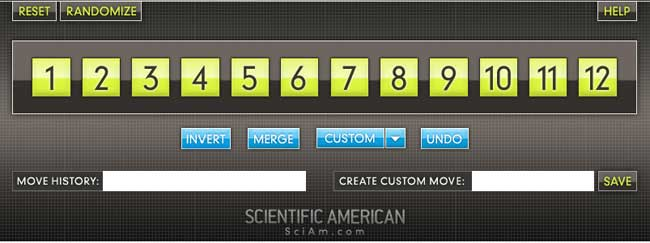Here is the help-screen :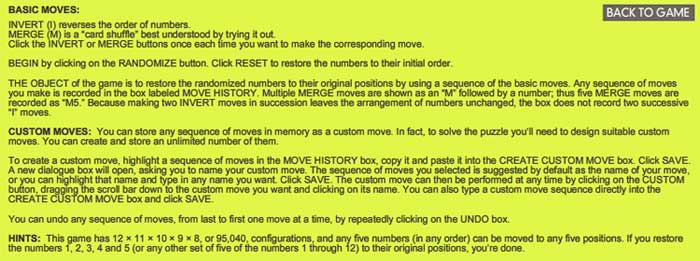They promise the solution by july 27th, but a few-line GAP-program cracks the puzzle instantly.

the M(24) game

Similar in nature, again using two generators of $M_{24}$. GAP-solution as before.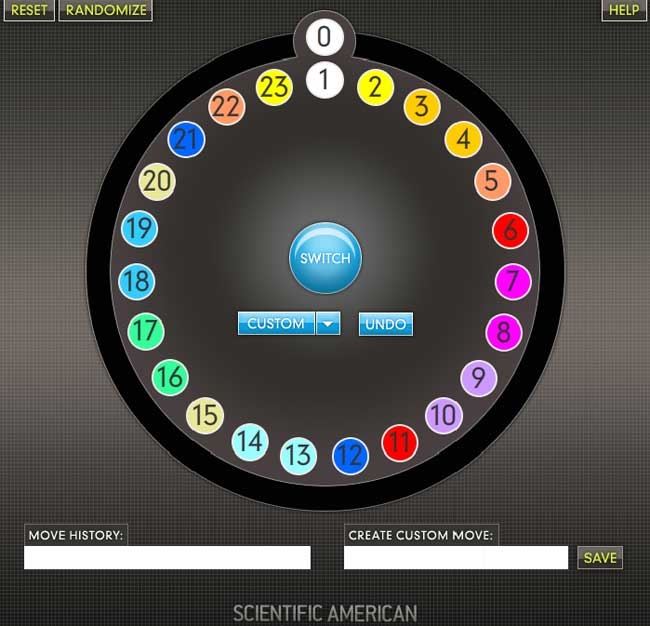This time, they offer this help-screen :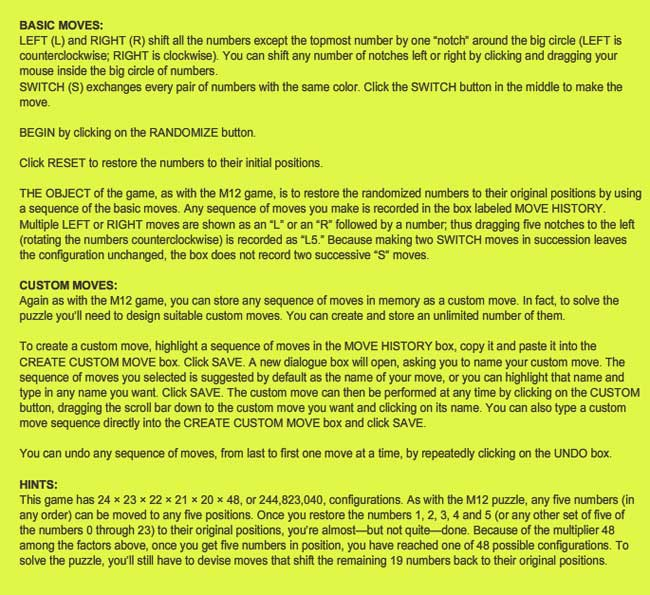the .0 game

Their most original game is based on Conway’s $.0$ (dotto) group. Unfortunately, they offer only a Windows-executable version, so I had to install Bootcamp and struggle a bit with taking screenshots on a MacBook to show you the game’s starting position :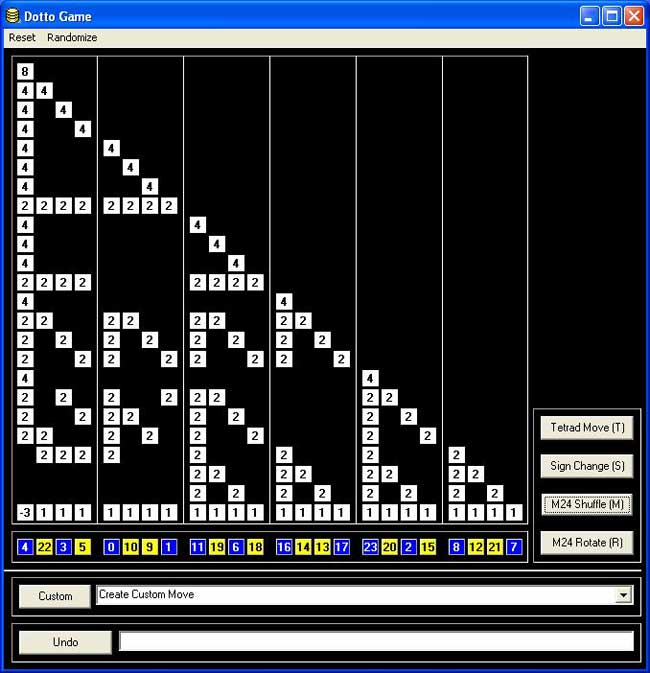Dotto:

Dotto, our final puzzle, represents the Conway group Co0, published in 1968 by mathematician John H. Conway of Princeton University. Co0 contains the sporadic simple group Co1 and has exactly twice as many members as Co1. Conway is too modest to name Co0 after himself, so he denotes the group “.0” (hence the pronunciation “dotto”).

In Dotto, there are four moves. This puzzle includes the M24 puzzle. Look at the yellow/blue row in the bottom. This is, in fact, M24, but the numbers are arranged in a row instead of a circle. The R move is the “circle rotation to the right”: the column above the number 0 stays put, but the column above the number 1 moves to the column over the number 2 etc. up to the column over the number 23, which moves to the column over the number 1. You may also click on a column number and then on another column number in the bottom row, and the “circle rotation” moving the first column to the second occurs. The M move is the switch, in each group of 4 columns separated by vertical lines (called tetrads) the “yellow” columns switch and the “blue” columns switch. The sign change move (S) changes signs of the first 8 columns (first two tetrads). The tetrad move (T) is the most complicated: Subtract in each row from each tetrad 1/2 times the sum of the numbers in that tetrad. Then in addition to that, reverse the signs of the columns in the first tetrad.

Strategy hints: Notice that the sum of squares of the numbers in each row doesn’t change. (This sum of squares is 64 in the first row, 32 in every other row.) If you manage to get an “8”in the first row, you have almost reduced the game to M24 except those signs. To have the original position, signs of all numbers on the diagonal must be +. Hint on signs: if the only thing wrong are signs on the diagonal, and only 8 signs are wrong, those 8 columns can be moved to the first 8 columns by using only the M24 moves (M,R).

Most chess programs are able to give a numerical evaluation of a position. For example, the position below is considered to be worth +8.7 with white to move, and, -0.7 with black to move (by a certain program). But, if one applies combinatorial game theory as in John Conway’s ONAG and the Berlekamp-Conway-Guy masterpiece Winning Ways for your Mathematical Plays it will turn out that the position can be proved to have an infinitesimal advantage for white…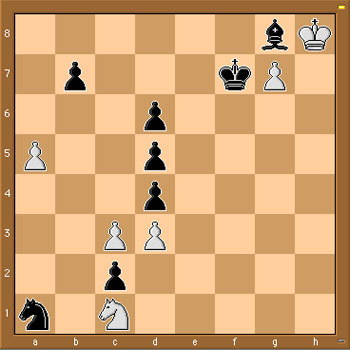So, what do we mean by this? First some basic rules of combinatorial game theory. To start, we evaluate a position without knowing which player has the move. A zero-game is by definition a position in which neither player has a good move, that is, any move by either player quickly leads to losing the game. Hence, a zero-game is a position in which the second player to move wins.

What is the chess-equivalent of a zero-position game? A position in which neither player has a good move is called a Mutual Zugzwang in chess literature. An example is given by the above position, if we restrict attention only to the 4 pieces in the upper right-hand corner and forget the rest. We don’t know who has the move, but, White cannot move at all and Black cannot move the King or Bishop without losing the Bishop and allowing White to promote the pawn and win quickly. In CGT-parlance, the upper-right position has value ${ \emptyset | \emptyset } = 0$ where the left options denote the White moves and the right options the Black moves.

All other values are determined by recursion. For example, consider a position in which White has just one move left before the sitution is again a Mutual Zugzwang, and, Black has no good move whatsoever. After white’s move, the position will again be a zero-position and Black has no options, so the value of this position would be denoted by ${ 0 | \emptyset }$ and we call the value of this position to be $+1$. Similarly, if white has no options and black has one final move to make, the position would be considered to have value ${ \emptyset | 0 }= -1$.

Clearly, these are just the three easiest game-values to have and the real kick comes further down the road when one can prove by recursion that some games have non-integer values (such as ${ 0 | 1 } = \frac{1}{2}$ for a position in which white has one move to get to a mutual zugzwang and black has a move leading to a position of value $+1$ (defined as before)), or non-number values such as $\ast = { 0 | 0 }$ where both white and black’s best move is to get to a mutal zugzwang. Game-values such as $\ast$ are called fuzzy (or confused with zero) and are defined by the property that the first player to move wins.

Similarly, positive game-values are those positions where White wins, independent of who has the move and negatives are those that Black wins. There is a whole menagery of game-values and the WinningWays-booklets give an example based introduction to this fascinating theory.

Brief as this introduction was, it will allow us to determine the exact value of the position in the above diagram. We know already that we can forget about the right-hand upper corner (as this is a zero-position) and concentrate attention to the left-hand side of the board.

It is easy to see that neither Knight can move without loosing quickly, nor can the pawns on a5 and b7. That is, white has just 2 options : either c3-c4 (quickly loosing after d5xc4 2. d3xc4,d4-d3 3. Nc1xd3,Na1-b3) or, and this is the only valid option c3xd4 leading to the position on the left below. Black has only one valid move : d4xc3 leading to the position on the right below.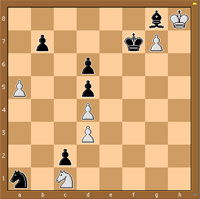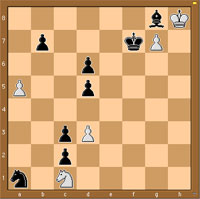Clearly, the left-diagram has value 0 as it is a mutual Zugzwang. The position on the right takes a moment’s thought : White has one move left d3-d4 leading to a 0-position, whereas black has one move d5-d4 leading to a position of value -1 (as black still has one move left d6-d5, whereas white has none). That is, the CGT-value of the right-hand position is ${ 0 | -1 }$ and therefore, the value of the starting position is precisely equal to

${ 0 | { 0 | -1 } } = +_{1}$ (called tiny-one among ONAGers)

It can be shown that $+_1$ has a positive value (that is, White wins independently of who has the first move) but smaller than any positive number-valued games!

Noam Elkies has written a beautiful paper On numbers and endgames: Combinatorial game theory in chess endgames containing many interesting examples (the example above is an adaptation of his diagram9).